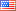# Revision history [back]

### Error when trying to integrate

f(x) = (1/3.33sqrt(2N(pi)))N(e)^((-1/2)((x-9.486)/3.33)^2)

When I do f.integrate(x,6,23) I get a big error.2 NoneJohn Palmieri6244 ●18 ●56 ●139 http://www.math.washin...

### Error when trying to integrate

With the function

f(x) = (1/3.33sqrt(2N(pi)))N(e)^((-1/2)((x-9.486)/3.33)^2)(1/3.33*sqrt(2*N(pi)))*N(e)^((-1/2)*((x-9.486)/3.33)^2)


When I do f.integrate(x,6,23) I get a big error.

### Error when trying to integrate

With the functionThis is what I want to do in Sage. It can be done very easily in Desmos. https://www.desmos.com/calculator/m6g...

f(x) = (1/3.33*sqrt(2*N(pi)))*N(e)^((-1/2)*((x-9.486)/3.33)^2)


I am just trying to do this integral in Sage. When I do it the answer I get is

When I do f.integrate(x,6,23) I get a big error.f(x) = (1/3.33sqrt(2pi))exp((-1/2)((x-9.486)/3.33)^2)

N(definite_integral(f(x), x, 6, 23))

5.35572539176154

### Error when trying to integrate

This is what I want to do in Sage. It can be done very easily in Desmos. https://www.desmos.com/calculator/m6g...https://www.desmos.com/calculator/7ocj57rixb

I am just trying to do this integral in Sage. When I do it the answer I get is

f(x) = (1/3.33sqrt(2pi))exp((-1/2)((x-9.486)/3.33)^2)

N(definite_integral(f(x), x, 6, 23))

5.35572539176154